# MySweeper Rules Version 1.08

### Symbols and Terms For Rules

Each cell is surrounded by at most eight other cells. Relative to these adjacent cells, each cell has associated with it the following fields:

#### Legend: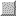= unexposed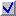= unexposed, but we know is a blank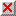= unexposed, but we know is a mine.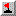= flagged as a mine

### Implemented Rules

MySweeper implements one-level rules, not transitive cases. That is, it doesn't act upon hints, only exposed cells and (possibly incorrectly) flagged cells. However, throughout this discussion, it is assumed that there are no incorrectly flagged cells.

 1. Prose: If an exposed cell has all its adjacent mines flagged, the remaining unexposed cells are empty. Technical: If for any exposed cell, ADJMINE == ADJFLAG, then none of the ADJUNEX cells are mines. Example: In the following configuration, the cells marked with aare known to be blank: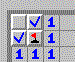2. Prose: If an unexposed cell has the same number of unexposed adjacent cells as there are unexposed mines, the remaining unexposed cells are all mines. Technical: If for any exposed cell, ADJMINE == (ADJFLAG + ADJUNEX), then all of the ADJUNEX cells are mines. Example: In the following configuration, the cells marked with aare known to be mines: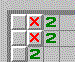3. Prose: If the unexposed cells surrounding cell #1 are a subset of the unexposed cells surrounding cell #2, and they have the same number of still buried (unflagged) mines, then none of the unexposed cells in R2 \ R1 are mines. Technical: Relative to the original cell(#1), for any exposed (within two cell radius) cells(#2) other than the current cell(#1) If (#(R2 INT R1) == #R1    && REST1 == REST2) Then all of the unexposed and unflagged cells in R2 \ R1 are blank. Example: In the following configuration, the cells marked with aare known to be blank: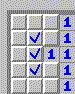4. Prose: If the still buried mines around cell #1 are fewer than the number of unexposed unflagged cells common to R2 and R1, and the difference between the number of unflagged mines between the two cells is the same as the number of unexposed unflagged cells in R2 \ R1, then all of the unexposed cells in R2 \ R1 are mines. Technical: Relative to the original cell(#1), for any exposed (within two cell radius) cells(#2) other than the current cell(#1) If (REST1 < #(R2 INT R1)    && (REST2 - REST1) == #(R2 \ R1)) Then all of the unexposed and unflagged cells in R2 \ R1 are mines and none of the unexposed and unflagged cells in R1 \ R2 are mines. Example: In the following configuration, the cells marked with aare known to be mines, and the cells marked with aare known to be blanks: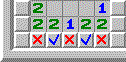### Unimplemented Rules

 5 The program does not as yet handle the following case: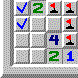In this case, the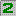on the bottom means that the twos to its left and above left contain one mine. This means that the twos above and to the above left of thealso contain one mine. Finally, theat the top left absorbs this last mine and thus the cells markedare blanks. To handle this, likely we need to recurse at each stage, or as has been suggested by Dave Rusin (), solve a set of simultaneous linear equations. 6 For the moment, no endgame rules have been implemented. For example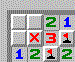If there's only one mine remaining, it's the cell marked. Using Dave Rusin's scheme this can be accomplished with one more simultaneous linear equation.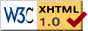© Sudley Place Software 1997-2019.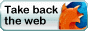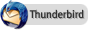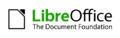Comments or suggestions? Send them to .